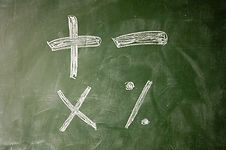A lot of curriculum time is devoted to developing addition and subtraction concepts and it is very important that younger pupils gain a firm understanding of these ideas at a young age so that they can build on this as they go through primary school.

Visuals

Using images and manipulatives helps pupils to represent the processes before moving on to more abstract calculations which is why they are widely found in classrooms of younger pupils. However, older primary pupils are sometimes expected to make the jump to abstract calculations rather suddenly since it can be dependent simply on which year group they have entered. The problem comes when pupils, who are still not confident, feel pressure to conform and are trying to work entirely in the abstract before they are ready.

Initially pupils use cubes, blocks and fingers (for calculations involving small numbers) as well as number tracks and the numbered number line that is usually displayed in classrooms.

Large number tracks and number lines can be used for pupils to jump along themselves and they can be easily created by joining pieces of paper, drawing chalk lines on the playground or even having some number lines painted as permanent markings on the playground. As pupils progress, blank number lines are useful for calculations and as a means of interpreting the problem visually.

As pupils progress, base ten materials can be used to illustrate the processes behind the algorithms used for addition and subtraction involving larger numbers.

Bar models are also excellent visual tools for helping pupils to understand addition and subtraction and can be used at all stages of primary school. They are also excellent for highlighting the links between addition and subtraction.

See Addition and Subtraction Facts in Resources, which uses bar models to help with assimilating addition and subtraction facts up to 10.

Working with addition and subtraction at the same time helps pupils to recognise the inverse relationship between addition and subtraction. Using bar models helps pupils to see the links between three numbers such as seven, three and ten.

7

3

10

Here, pupils can see that putting seven and three together results in ten,   whilst removing three from ten results in seven and removing seven from ten results in three. This can first be represented using interlocking cubes but can then be applied to any size number and helps pupils to see that when solving subtraction calculations, we can ask what we need to add to get to the original number.

It also allows us to check subtraction problems by using addition, which is not always obvious to pupils. Missing number problems also benefit from this approach since 7 + ? = 10 can be represented by the bar model above, where the numeral 3 would be replaced by the symbol ?

## Once we have an idea of the magnitude, a simple calculation involving the last digit of each amount can give us another indication as to how likely the answer is to be correct.

Pupils who have not been encouraged to estimate when calculating are more likely to produce solutions which do not make any sense.

## ​

See Resources for a download of how to use SPOT when working on context problems in your classroom.

## Language

We need to be careful in our use of language in maths classes since we can assume that pupils understand the language we are using, unlike in English, where we are far more likely to explore the language as we are reading text. We can also inadvertently confuse pupils when we use words that have a different meaning in maths than they do when used in other situations.

Sums is an example of a word that is used (particularly by older adults) to refer to all forms of arithmetic when it should be reserved only for addition - sum means to add.

Difference confuses some pupils who think, 'but they're both the same - they're both numbers, so there is no difference...'

## Emphasising the use of equals and the idea of a balance when working with number systems can help to avoid this.

Provide pupils with specific words and ask them to see how many different types of problems can be created in pairs, using the given words. This is a useful exercise for highlighting language that needs to be worked on.## Fluency

It is important that pupils can calculate effectively and confidently and one way to approach this is to provide pupils with methods, followed by lots of practice. However, if pupils have developed number sense, this allows them to be far more flexible when working with numbers.

An example of this would be when working with 18 - 9, which can be seen as 18 - 10 and adjust by one or it can be seen as the difference between 18 and 9. When we look at this on a number line, this is the same as 19 - 10 or 20 - 11 (amongst others).

When applied to larger numbers, this flexibility can be very effective: 178 - 39 can be seen as 179 - 40 while 1024 + 791 is the same as 1015 + 9 + 791 which becomes 1015 + 800.

When carrying out subtraction algorithms, such as 2001 - 278, it is helpful if the pupils understand that 2001 can be written as 1900 + 100 + 1 which becomes 1900 + 90 + 11.

Encourage reasoning when playing with the numbers in calculations, such as: 194 - 87 is easier as 200 - 93 (adding six to both numbers) but why can we add the same amount to each number for subtraction but 97 + 84 becomes 100 + 81? This can result in an interesting discussion, using number lines to explain their thoughts.